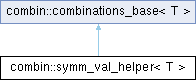Cadabra Computer algebra system for field theory problems
combin::symm_val_helper< T > Class Template Reference

#include <Combinatorics.hh>

Inheritance diagram for combin::symm_val_helper< T >:## Public Member Functions

symm_val_helper (symmetriser< T > &)

virtual void clear ()Public Member Functions inherited from combin::combinations_base< T >
combinations_base ()

combinations_base (const std::vector< T > &)

virtual ~combinations_base ()

void permute (long start=-1, long end=-1)

virtual void clear_results ()

unsigned int sum_of_sublengths () const

void set_unit_sublengths ()

unsigned int multiplier (const std::vector< T > &) const

unsigned int total_permutations () const

## Public Attributes

int current_multiplicityPublic Attributes inherited from combin::combinations_base< T >
unsigned int block_length

std::vector< unsigned int > sublengths

range_vector_t input_asym

std::vector< T > original

bool multiple_pick

std::vector< weights_tweights

std::vector< int > max_weights

std::vector< weight_condweight_conditions

unsigned int sub_problem_blocksize

## Protected Member Functions

virtual void vector_generated (const std::vector< unsigned int > &)Protected Member Functions inherited from combin::combinations_base< T >
virtual bool entry_accepted (unsigned int current) const

## Protected Attributes

bool first_one

symmetriser< T > & owner_Protected Attributes inherited from combin::combinations_base< T >
std::vector< unsigned int > temparr

long start_

long end_

long vector_generated_called_

std::vector< int > current_weightPublic Types inherited from combin::combinations_base< T >
enum  weight_cond { weight_equals, weight_less, weight_greater }

## ◆ symm_val_helper()

template<class T >
 combin::symm_val_helper< T >::symm_val_helper ( symmetriser< T > & tt )

## ◆ clear()

template<class T >
 void combin::symm_val_helper< T >::clear
virtual

Reimplemented from combin::combinations_base< T >.

## ◆ vector_generated()

template<class T >
 void combin::symm_val_helper< T >::vector_generated ( const std::vector< unsigned int > & vec )
protectedvirtual

## ◆ current_multiplicity

template<class T >
 int combin::symm_val_helper< T >::current_multiplicity

## ◆ first_one

template<class T >
 bool combin::symm_val_helper< T >::first_one
protected

## ◆ owner_

template<class T >
 symmetriser& combin::symm_val_helper< T >::owner_
protected

The documentation for this class was generated from the following file: